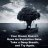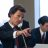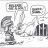# Futures Market Ratio Indicator for ThinkorSwim#### BenTen

Staff
VIP
Warehouse
This one is very useful for futures traders on 15 min and daily. The way it is set now is /RTY vs /ZN because I trade /RTY and /ES, but it is a ratio indicator, so it can be set to any ratio. Generally speaking, when bonds go up index futures go down. I also use it to see a ration of /ES vs /VX. It can probably be improved with a simple moving average, because as is it is using the high/low of a 20 MA.### thinkScript Code

Rich (BB code):
``````declare lower;

input secondSym = "/ZN";
def div = if !IsNaN (close("/RTY")) then close("/RTY") else div;
def RatioQ = close (secondSym) / div;
plot Ratio = RatioQ;

#def divZ = if !IsNaN (close("/RTY")) then close("/RTY") else divZ;
#def RatioZ = close("/ZB") / divZ;
#plot RatioZB = RatioZ;

def RatioQH = high (secondSym) / div;
plot RatioH = RatioQH;

def RatioQL = low (secondSym) / div;
plot RatioL = RatioQL;

AddVerticalLine(if Ratio crosses above RatioH then 1 else 0, "S", Color.RED);
AddVerticalLine(if Ratio crosses below RatioL then 1 else 0, "B", Color.GREEN);

#(low(secondSym))

#plot ave = data;
#ave.SetLineWeight(2);

Ratio.DefineColor("Up", Color.Green);
Ratio.DefineColor("Down", Color.Red);
Ratio.DefineColor("Equal", Color.Yellow);
Ratio.AssignValueColor
(
if Ratio > Ratio
then Ratio.color("Up")
else if Ratio < Ratio
then Ratio.color("Down")
else Ratio.color("Equal")
);``````

A slightly different version of the above ratio:

Thanks @john3 for sending in the code.

Last edited:
•markos#### markos

##### Well-known member
VIP
Warehouse
Very nice, @john3 , Thanks @BenTen !

•BenTenQuestions 3Questions 2Index Futures 5